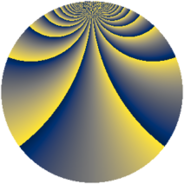# Properties

 Label 1140.2.cgLevel $1140$ Weight $2$ Character orbit 1140.cg Rep. character $\chi_{1140}(131,\cdot)$ Character field $\Q(\zeta_{18})$ Dimension $960$ Sturm bound $480$

# Related objects

## Defining parameters

 Level: $$N$$ $$=$$ $$1140 = 2^{2} \cdot 3 \cdot 5 \cdot 19$$ Weight: $$k$$ $$=$$ $$2$$ Character orbit: $$[\chi]$$ $$=$$ 1140.cg (of order $$18$$ and degree $$6$$) Character conductor: $$\operatorname{cond}(\chi)$$ $$=$$ $$228$$ Character field: $$\Q(\zeta_{18})$$ Sturm bound: $$480$$

## Dimensions

The following table gives the dimensions of various subspaces of $$M_{2}(1140, [\chi])$$.

Total New Old
Modular forms 1488 960 528
Cusp forms 1392 960 432
Eisenstein series 96 0 96

## Trace form

 $$960q + 6q^{4} + 12q^{9} + O(q^{10})$$ $$960q + 6q^{4} + 12q^{9} + 6q^{10} + 24q^{13} - 6q^{16} - 24q^{30} - 12q^{33} - 42q^{34} + 120q^{36} + 186q^{42} + 66q^{48} + 480q^{49} - 48q^{52} - 66q^{54} + 48q^{61} - 42q^{64} + 60q^{66} - 72q^{70} - 84q^{72} + 216q^{73} - 108q^{76} + 60q^{78} - 60q^{81} + 96q^{85} - 48q^{88} - 54q^{90} - 24q^{94} - 252q^{96} + 72q^{97} + O(q^{100})$$

## Decomposition of $$S_{2}^{\mathrm{new}}(1140, [\chi])$$ into newform subspaces

The newforms in this space have not yet been added to the LMFDB.

## Decomposition of $$S_{2}^{\mathrm{old}}(1140, [\chi])$$ into lower level spaces

$$S_{2}^{\mathrm{old}}(1140, [\chi]) \cong$$ $$S_{2}^{\mathrm{new}}(228, [\chi])$$$$^{\oplus 2}$$